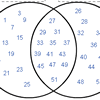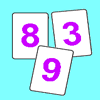# Resources tagged with: Prime numbers

Filter by: Content type:
Age range:
Challenge level:

### There are 25 results

Broad Topics > Properties of Numbers > Prime numbers### Tom's Number

##### Age 7 to 11 Challenge Level:

Work out Tom's number from the answers he gives his friend. He will only answer 'yes' or 'no'.### Powerful Factorial

##### Age 11 to 14 Challenge Level:

6! = 6 x 5 x 4 x 3 x 2 x 1. The highest power of 2 that divides exactly into 6! is 4 since (6!) / (2^4 ) = 45. What is the highest power of two that divides exactly into 100!?### Sieve of Eratosthenes

##### Age 11 to 14 Challenge Level:

Follow this recipe for sieving numbers and see what interesting patterns emerge.### One to Eight

##### Age 11 to 14 Challenge Level:

Complete the following expressions so that each one gives a four digit number as the product of two two digit numbers and uses the digits 1 to 8 once and only once.### Just Repeat

##### Age 11 to 14 Challenge Level:

Think of any three-digit number. Repeat the digits. The 6-digit number that you end up with is divisible by 91. Is this a coincidence?### Factoring Factorials

##### Age 11 to 14 Challenge Level:

Find the highest power of 11 that will divide into 1000! exactly.### Thirty Six Exactly

##### Age 11 to 14 Challenge Level:

The number 12 = 2^2 � 3 has 6 factors. What is the smallest natural number with exactly 36 factors?### Code Breaker

##### Age 7 to 11 Challenge Level:

This problem is based on a code using two different prime numbers less than 10. You'll need to multiply them together and shift the alphabet forwards by the result. Can you decipher the code?### Factors and Multiples Game for Two

##### Age 7 to 14 Challenge Level:

Factors and Multiples game for an adult and child. How can you make sure you win this game?### Stars

##### Age 11 to 14 Challenge Level:

Can you find a relationship between the number of dots on the circle and the number of steps that will ensure that all points are hit?### A Conversation Piece

##### Age 7 to 11 Challenge Level:

Take the number 6 469 693 230 and divide it by the first ten prime numbers and you'll find the most beautiful, most magic of all numbers. What is it?### Flow Chart

##### Age 11 to 14 Challenge Level:

The flow chart requires two numbers, M and N. Select several values for M and try to establish what the flow chart does.### Factor Lines

##### Age 7 to 14 Challenge Level:

Arrange the four number cards on the grid, according to the rules, to make a diagonal, vertical or horizontal line.### Various Venns

##### Age 7 to 11 Challenge Level:

Use the interactivities to complete these Venn diagrams.##### Age 11 to 14 Challenge Level:

Great Granddad is very proud of his telegram from the Queen congratulating him on his hundredth birthday and he has friends who are even older than he is... When was he born?### Gaxinta

##### Age 11 to 14 Challenge Level:

A number N is divisible by 10, 90, 98 and 882 but it is NOT divisible by 50 or 270 or 686 or 1764. It is also known that N is a factor of 9261000. What is N?### Two Primes Make One Square

##### Age 7 to 11 Challenge Level:

Can you make square numbers by adding two prime numbers together?### Factors and Multiples Puzzle

##### Age 11 to 14 Challenge Level:

Using your knowledge of the properties of numbers, can you fill all the squares on the board?### Factors and Multiples Game

##### Age 7 to 16 Challenge Level:

A game in which players take it in turns to choose a number. Can you block your opponent?### Prompt Cards

##### Age 7 to 11 Challenge Level:

These two group activities use mathematical reasoning - one is numerical, one geometric.### Penta Primes

##### Age 7 to 11 Challenge Level:

Using all ten cards from 0 to 9, rearrange them to make five prime numbers. Can you find any other ways of doing it?### Round and Round the Circle

##### Age 7 to 11 Challenge Level:

What happens if you join every second point on this circle? How about every third point? Try with different steps and see if you can predict what will happen.### Strange Numbers

##### Age 11 to 14 Challenge Level:

All strange numbers are prime. Every one digit prime number is strange and a number of two or more digits is strange if and only if so are the two numbers obtained from it by omitting either. . . .### Swimming Pool Tiles

##### Age 7 to 11 Challenge Level:

This activity creates an opportunity to explore all kinds of number-related patterns.### Coins

##### Age 11 to 14 Challenge Level:

A man has 5 coins in his pocket. Given the clues, can you work out what the coins are?